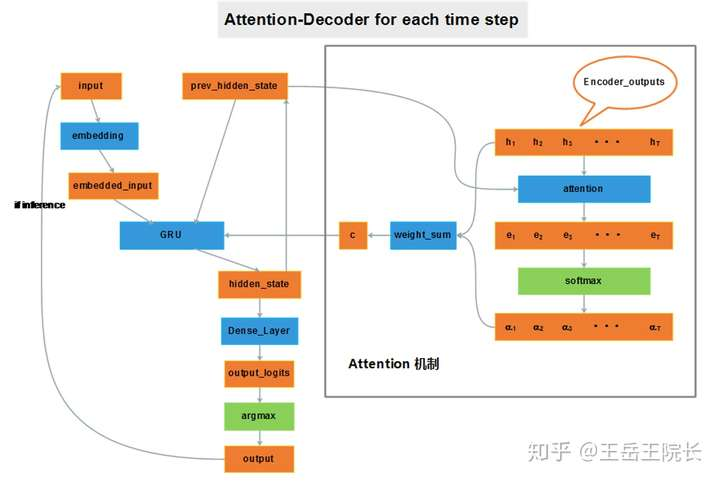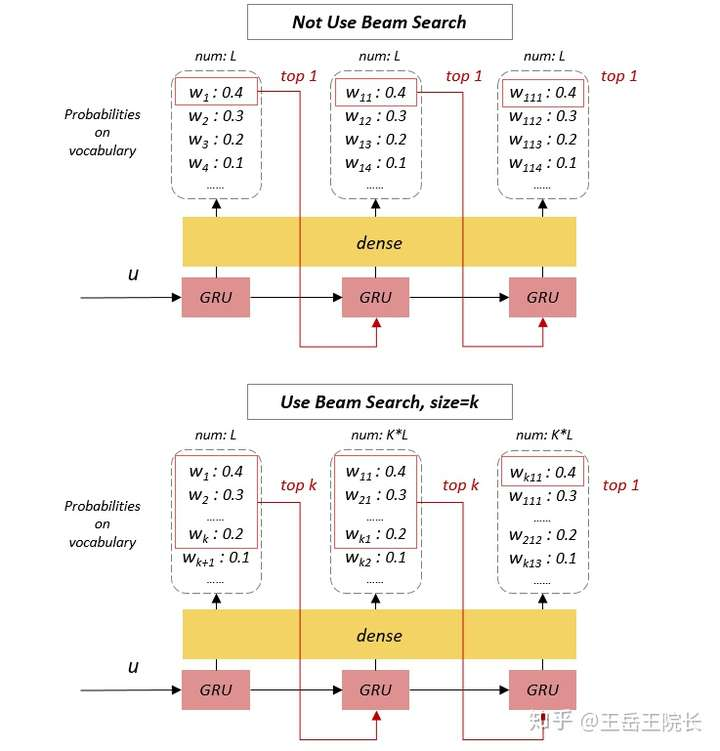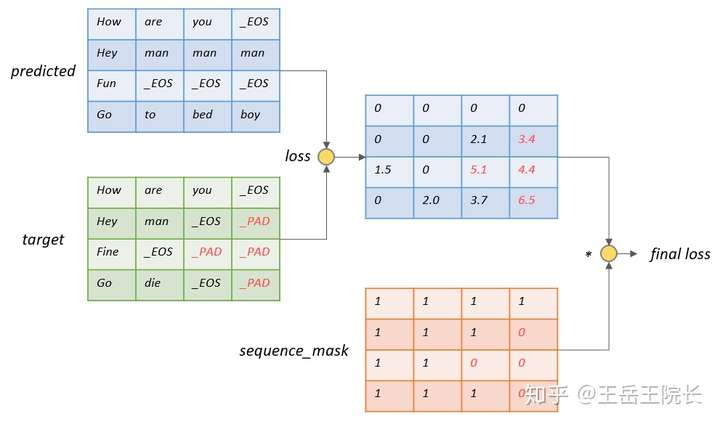# Tensorflow中的Seq2Seq全家桶

2019-12-03 09:53:41  阅读：51  来源： 互联网

Seq2Seq 模型顾名思义，输入一个序列，用一个 RNN （Encoder）编码成一个向量 u，再用另一个 RNN （Decoder）解码成一个序列输出，且输出序列的长度是可变的。用途很广，机器翻译，自动摘要，对话系统，还有上一篇文章里我用来做多跳问题的问答，只要是序列对序列的问题都能来搞，功能很强大，效果也不错。```import tensorflow as tf

class Seq2seq(object):
def __init__(self, config, w2i_target):
self.seq_inputs = tf.placeholder(shape=(config.batch_size, None), dtype=tf.int32, name='seq_inputs')
self.seq_inputs_length = tf.placeholder(shape=(config.batch_size,), dtype=tf.int32, name='seq_inputs_length')
self.seq_targets = tf.placeholder(shape=(config.batch_size, None), dtype=tf.int32, name='seq_targets')
self.seq_targets_length = tf.placeholder(shape=(config.batch_size,), dtype=tf.int32, name='seq_targets_length')

with tf.variable_scope("encoder"):
encoder_embedding = tf.Variable(tf.random_uniform([config.source_vocab_size, config.embedding_dim]), dtype=tf.float32, name='encoder_embedding')
encoder_inputs_embedded = tf.nn.embedding_lookup(encoder_embedding, self.seq_inputs)
encoder_cell = tf.nn.rnn_cell.GRUCell(config.hidden_dim)
encoder_outputs, encoder_state = tf.nn.dynamic_rnn(cell=encoder_cell, inputs=encoder_inputs_embedded, sequence_length=self.seq_inputs_length, dtype=tf.float32, time_major=False)

tokens_go = tf.ones([config.batch_size], dtype=tf.int32) * w2i_target["_GO"]
decoder_inputs = tf.concat([tf.reshape(tokens_go,[-1,1]), self.seq_targets[:,:-1]], 1)

with tf.variable_scope("decoder"):
decoder_embedding = tf.Variable(tf.random_uniform([config.target_vocab_size, config.embedding_dim]), dtype=tf.float32, name='decoder_embedding')
decoder_inputs_embedded = tf.nn.embedding_lookup(decoder_embedding, decoder_inputs)
decoder_cell = tf.nn.rnn_cell.GRUCell(config.hidden_dim)
decoder_outputs, decoder_state = tf.nn.dynamic_rnn(cell=decoder_cell, inputs=decoder_inputs_embedded, initial_state=encoder_state, sequence_length=self.seq_targets_length, dtype=tf.float32, time_major=False)

decoder_logits = tf.layers.dense(decoder_outputs.rnn_output, config.target_vocab_size)
self.out = tf.argmax(decoder_logits, 2)```## 1. Teacher Forcing1. 防止上一时刻的错误传播到这一时刻，decode 出一个序列，要是第一个单词错了，整个序列就跑偏了，这个序列就没啥意义了，计算 loss 更新参数作用都很小了。用了 Teacher Forcing 可以阻断错误积累，斧正模型训练，加快参数收敛（我自己试了一下，用和不用 Teacher Forcing，训练时候的 loss 下降速度和最终结果真的差了不少）
2. 这样就可以提前把 decoder 的整个输入序列提前准备好，直接放到 dynamic_rnn 函数就能出结果，实现起来简单方便

（算了不放了，20多行太长了。总之很麻烦，感兴趣可以去源代码里的 model_seq2seq.py 看一下）

```tokens_go = tf.ones([config.batch_size], dtype=tf.int32) * w2i_target["_GO"]
decoder_embedding = tf.Variable(tf.random_uniform([config.target_vocab_size, config.embedding_dim]), dtype=tf.float32, name='decoder_embedding')
decoder_cell = tf.nn.rnn_cell.GRUCell(config.hidden_dim)
if useTeacherForcing:
decoder_inputs = tf.concat([tf.reshape(tokens_go,[-1,1]), self.seq_targets[:,:-1]], 1)
helper =tf.contrib.seq2seq.TrainingHelper(tf.nn.embedding_lookup(decoder_embedding, decoder_inputs), self.seq_targets_length)
else:
helper = tf.contrib.seq2seq.GreedyEmbeddingHelper(decoder_embedding, tokens_go, w2i_target["_EOS"])
decoder = tf.contrib.seq2seq.BasicDecoder(decoder_cell, helper, encoder_state, output_layer=tf.layers.Dense(config.target_vocab_size))
decoder_outputs, decoder_state, final_sequence_lengths = tf.contrib.seq2seq.dynamic_decode(decoder, maximum_iterations=tf.reduce_max(self.seq_targets_length))```

dynamic_decode 函数类似于 dynamic_rnn，帮你自动执行 rnn 的循环，返回完整的输出序列

## 2. Attention

seq2seq 里 attention 的作用就不详细说了，直接放一个我看到过的最直观的一个图，图片来源写在图注里，侵删。图片来源：CSDN上博主thriving_fcl的博客，https://blog.csdn.net/thriving_fcl/article/details/74853556

``````decoder_cell = tf.nn.rnn_cell.GRUCell(config.hidden_dim)
if useAttention:
attention_mechanism = tf.contrib.seq2seq.BahdanauAttention(num_units=config.hidden_dim, memory=encoder_outputs, memory_sequence_length=self.seq_inputs_length)
# attention_mechanism = tf.contrib.seq2seq.LuongAttention(num_units=config.hidden_dim, memory=encoder_outputs, memory_sequence_length=self.seq_inputs_length)
decoder_cell = tf.contrib.seq2seq.AttentionWrapper(decoder_cell, attention_mechanism)
decoder_initial_state = decoder_cell.zero_state(batch_size=config.batch_size, dtype=tf.float32)
decoder_initial_state = decoder_initial_state.clone(cell_state=encoder_state)

decoder = tf.contrib.seq2seq.BasicDecoder(decoder_cell, helper, decoder_initial_state, output_layer=tf.layers.Dense(config.target_vocab_size))
decoder_outputs, decoder_state, final_sequence_lengths = tf.contrib.seq2seq.dynamic_decode(decoder, maximum_iterations=tf.reduce_max(self.seq_targets_length))``````

``````def attn(self, hidden, encoder_outputs):
# hidden: B * D
# encoder_outputs: B * S * D
attn_weights = tf.matmul(encoder_outputs, tf.expand_dims(hidden, 2))
# attn_weights: B * S * 1
attn_weights = tf.nn.softmax(attn_weights, axis=1)
context = tf.squeeze(tf.matmul(tf.transpose(encoder_outputs, [0,2,1]), attn_weights))
# context: B * D
return context
# ……
input = tf.cond(finished, lambda: tokens_eos_embedded, get_next_input)
if useAttention:
input = tf.concat([input, self.attn(previous_state, encoder_outputs)], 1)
# ……``````

## 3. Beam Search``````tokens_go = tf.ones([config.batch_size], dtype=tf.int32) * w2i_target["_GO"]
decoder_cell = tf.nn.rnn_cell.GRUCell(config.hidden_dim)

if useBeamSearch > 1:
decoder_initial_state = tf.contrib.seq2seq.tile_batch(encoder_state, multiplier=useBeamSearch)
decoder = tf.contrib.seq2seq.BeamSearchDecoder(decoder_cell, decoder_embedding, tokens_go, w2i_target["_EOS"],  decoder_initial_state , beam_width=useBeamSearch, output_layer=tf.layers.Dense(config.target_vocab_size))
else:
decoder_initial_state = encoder_state
decoder = tf.contrib.seq2seq.BasicDecoder(decoder_cell, helper, decoder_initial_state, output_layer=tf.layers.Dense(config.target_vocab_size))

decoder_outputs, decoder_state, final_sequence_lengths = tf.contrib.seq2seq.dynamic_decode(decoder, maximum_iterations=tf.reduce_max(self.seq_targets_length))``````

## 4. Sequence Loss``````sequence_mask = tf.sequence_mask(self.seq_targets_length, dtype=tf.float32)

``````sequence_mask = tf.sequence_mask(self.seq_targets_length, dtype=tf.float32)
loss = tf.nn.sparse_softmax_cross_entropy_with_logits(logits=decoder_logits, labels=self.seq_targets)

## 最后再上一遍结论吧

tensorflow 所提供的这个 seq2seq 全家桶功能还是很强大，很多比如 Beam Search 这些实现起来需要弯弯绕绕写一大段，很麻烦的事情，直接调个接口，一句话就能用，省时省力，很nice